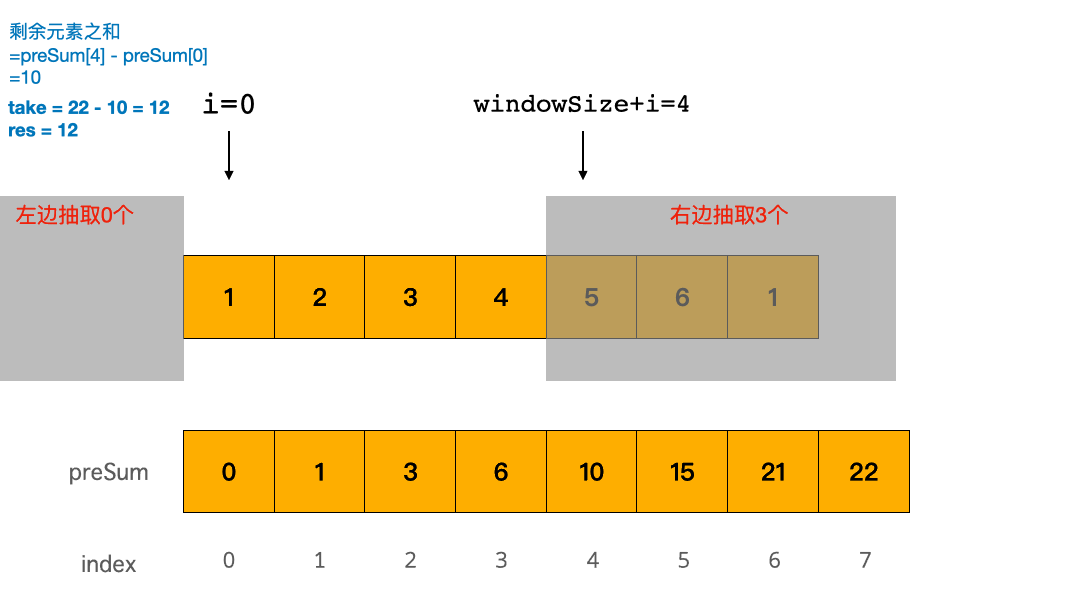# 1423. Maximum Points You Can Obtain from Cards 可获得的最大点数

• 作者： 负雪明烛
• id： fuxuemingzhu
• 公众号：负雪明烛
• 本文关键词：LeetCode，力扣，算法，算法题，滑动窗口，递归，前缀和，preSum，刷题群

@TOC

# # 题目描述

``````输入：cardPoints = [1,2,3,4,5,6,1], k = 3

``````

``````输入：cardPoints = [2,2,2], k = 2

``````

``````输入：cardPoints = [9,7,7,9,7,7,9], k = 7

``````

``````输入：cardPoints = [1,1000,1], k = 1

``````

``````输入：cardPoints = [1,79,80,1,1,1,200,1], k = 3

``````

• `1 <= cardPoints.length <= 10^5`
• `1 <= cardPoints[i] <= 10^4`
• `1 <= k <= cardPoints.length`

# # 解题思路

## # 递归

``````class Solution(object):
def maxScore(self, cardPoints, k):
"""
:type cardPoints: List[int]
:type k: int
:rtype: int
"""
N = len(cardPoints)
self.memo = {}
return self.dfs(cardPoints, 0, N - 1, k)

def dfs(self, cardPoints, i, j, k):
if k == 0:
return 0
if (i, j) in self.memo:
return self.memo[(i, j)]
removeLeft = cardPoints[i] + self.dfs(cardPoints, i + 1, j, k - 1)
removeRight = cardPoints[j] + self.dfs(cardPoints, i, j - 1, k - 1)
res = max(removeLeft, removeRight)
self.memo[(i, j)] = res
return res
``````

## # preSum

``````N = len(nums)
preSum = range(N + 1)
for i in range(N):
preSum[i + 1] = preSum[i] + nums[i]
print(preSum)
``````

sum(i, j) = preSum[i + 1] - preSum[j]``````class Solution(object):
def maxScore(self, cardPoints, k):
"""
:type cardPoints: List[int]
:type k: int
:rtype: int
"""
N = len(cardPoints)
preSum =  * (N + 1)
for i in range(N):
preSum[i + 1] = preSum[i] + cardPoints[i]
res = float("inf")
windowSize = N - k
for i in range(k + 1):
res = min(res, preSum[windowSize + i] - preSum[i])
return preSum[N] - res
``````

## # 滑动窗口

• `i >= windowSize` 时，为了固定窗口的元素是 `k` 个，每次移动时需要将 `i - windowSize` 位置的元素移除。
• `i >= windowSize - 1` 时，滑动窗口内的元素刚好是 `k` 个，开始计算滑动窗口的最小和

``````class Solution(object):
def maxScore(self, cardPoints, k):
"""
:type cardPoints: List[int]
:type k: int
:rtype: int
"""
N = len(cardPoints)
windowSize = N - k # 窗口的大小
sums = 0
res = float("inf") # 正无穷大
for i in range(N):
sums += cardPoints[i]
if i >= windowSize:
sums -= cardPoints[i - windowSize]
if i >= windowSize - 1:
res = min(res, sums)
return sum(cardPoints) - res
``````

# # 刷题心得

1. 今天这个题目，难点还是需要把抽取卡牌的问题转变为一个滑动窗口的问题。
2. 问题抽象之后，preSum 和 滑动窗口 两种解法就已经呼之欲出了。
3. 每日一题的作用再次凸显，同一类题目都是换汤不换药，学会思路和套路之后，就很快秒杀！

# # 欢迎加入组织# # 日期

2021 年 2 月 6 日 —— 今天是年前的最后一个假期，没剪上头发